Author: Oscar Cronquist Article last updated on May 10, 2022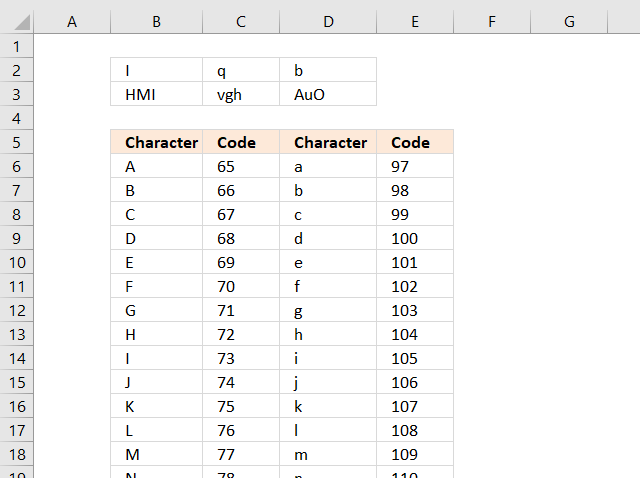The RAND() function In Excel returns a number greater than or equal to 0 (zero) and less than 1. Combining the RAND() function with a few other functions makes it possible to create random numbers, text, dates and time values.

I will in this article demonstrate what they do and how these formulas work. Update! The RANDBETWEEN function is in many cases a better and easier function, I have added formulas for this option as well.

### 1. Random numbers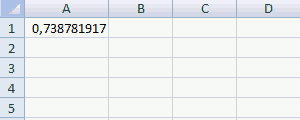This example demonstrates the RAND function returning a number greater than or equal to 0 (zero) and less than 1 each time a worksheet is recalculated.

Formula in cell A1:

=RAND()

Press F9 to recalculate all sheets in all open workbooks, this will also create a new random number in cell A1, see animated image above.

Note, the RAND function is a volatile function meaning it is recalculated each time the worksheet is recalculated. This may slow down your worksheet considerably if you use a lot of these functions.

1.1 How can we use this function to return numbers between or equal to 0 and 100?

Formula in cell A3:

=ROUNDUP(RAND()*101, 0)-1

ROUNDUP(number, num_digits) rounds a number up, away from zero.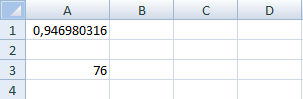The RANDBETWEEN function is a better choice in this case.

=RANDBETWEEN(1, 100)

#### 1.2 How to return numbers between or equal to -50 and 50?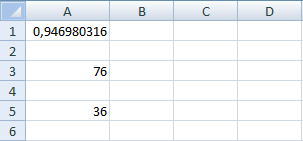Formula in cell A5:

=ROUNDUP(RAND()*101, 0)-51

ROUNDUP(number, num_digits) rounds a number up, away from zero.

If you use the RANDBETWEEN function the formula becomes:

=RANDBETWEEN(-50,50)

### 2. Random text stringsA character in Excel is represented by a number based on the character set, in windows ANSI. A to Z are 65 to 90 and a to z are 97 to 122.

=CHAR(number) returns the character specified by the code number from the character set of your computer.

You can use the CHAR, RAND and ROUNDUP function to return random characters, now let me show you how.

#### 2.1 A random uppercase letter

The following formula returns a single random uppercase letter from A to Z.

Formula in B2:

=CHAR(ROUNDUP(RAND()*26,0)-1+65)

or use the RANDBETWEEN function which makes the formula smaller and easier to understand.

=CHAR(RANDBETWEEN(65, 90))

#### 2.2 A random lowercase letter

The following formula returns a single random lowercase letter from a to z.

Formula in C2:

=CHAR(ROUNDUP(RAND()*26,0)-1+97)

or a better formula:

=CHAR(RANDBETWEEN(97, 122))

#### 2.3 A random lowercase or uppercase letter

This formula returns a random lowercase or uppercase letter.

Formula in D2:

=IF(RAND()<0.5, CHAR(ROUNDUP(RAND()*26, 0)-1+65), CHAR(ROUNDUP(RAND()*26, 0)-1+97))

A formula based on the RANDBETWEEN function:

=IF(RAND()<0.5, CHAR(RANDBETWEEN(65, 90)),CHAR(RANDBETWEEN(97, 122))

#### 2.4 Three random uppercase letters

This formula concatenates three random uppercase letter A to Z.

Formula in B3:

=CHAR(ROUNDUP(RAND()*26, 0)-1+65)&CHAR(ROUNDUP(RAND()*26, 0)-1+65)&CHAR(ROUNDUP(RAND()*26, 0)-1+65)

A formula based on the RANDBETWEEN function:

=CHAR(RANDBETWEEN(65, 90))&CHAR(RANDBETWEEN(65, 90))&CHAR(RANDBETWEEN(65, 90))

#### 2.5 Three random lowercase letters

This formula concatenates three random lowercase letter a to z.

Formula in C3

=CHAR(ROUNDUP(RAND()*26, 0)-1+97)&CHAR(ROUNDUP(RAND()*26, 0)-1+97)&CHAR(ROUNDUP(RAND()*26, 0)-1+97)

A formula based on the RANDBETWEEN function:

=CHAR(RANDBETWEEN(97, 122))&CHAR(RANDBETWEEN(97, 122))&CHAR(RANDBETWEEN(97, 122))

#### 2.6 Three random lowercase and uppercase letters

The forllowing formula returns three random letters that may be lowercase or uppercase.

Formula in D3:

=IF(RAND()<0.5, CHAR(ROUNDUP(RAND()*26, 0)-1+65), CHAR(ROUNDUP(RAND()*26, 0)-1+97))&IF(RAND()<0.5, CHAR(ROUNDUP(RAND()*26, 0)-1+65), CHAR(ROUNDUP(RAND()*26, 0)-1+97))&IF(RAND()<0.5, CHAR(ROUNDUP(RAND()*26, 0)-1+65), CHAR(ROUNDUP(RAND()*26, 0)-1+97))

A formula based on the RANDBETWEEN function:

=IF(RAND()<0.5, CHAR(RANDBETWEEN(65, 90)), CHAR(RANDBETWEEN(97, 122)))&IF(RAND()<0.5, CHAR(RANDBETWEEN(65, 90)), CHAR(RANDBETWEEN(97, 122)))&IF(RAND()<0.5, CHAR(RANDBETWEEN(65, 90)), CHAR(RANDBETWEEN(97, 122)))

#### Explaining RANDBETWEEN formula in cell D3

Step 1 - Create  arandom number between 0 and 1

The RAND function has no arguments, it simply returns a number between 0 and 1.

RAND()

returns 0.377261423327488 (random number)

Step 2 - If random number is smaller than 0.5

The IF function checks if the logical expression RAND()<0.5 is TRUE or FALSE, if TRUE one thing happens in the second argument and if FALSE another thing happens in the third argument.

IF(RAND()<0.5, CHAR(RANDBETWEEN(65, 90)), CHAR(RANDBETWEEN(97, 122)))

becomes

IF(0.377261423327488<0.5 , CHAR(RANDBETWEEN(65, 90)), CHAR(RANDBETWEEN(97, 122)))

becomes

IF(TRUE , CHAR(RANDBETWEEN(65, 90)), CHAR(RANDBETWEEN(97, 122)))

becomes

CHAR(RANDBETWEEN(65, 90))

and returns a random uppercase letter.

Step 3 - Concatenate characters

The ampersand character concatenates the upper and lower case letter

IF(RAND()<0.5, CHAR(RANDBETWEEN(65, 90)), CHAR(RANDBETWEEN(97, 122)))&IF(RAND()<0.5, CHAR(RANDBETWEEN(65, 90)), CHAR(RANDBETWEEN(97, 122)))&IF(RAND()<0.5, CHAR(RANDBETWEEN(65, 90)), CHAR(RANDBETWEEN(97, 122)))

may become "X"&"h"&"A"

and returns XhA in cell D3.

### 3. Random dates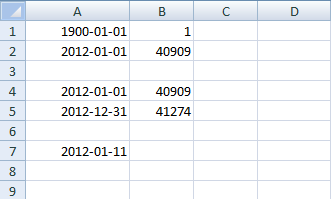Excel uses numbers as dates, called the 1900-system. The earliest date you can use is 1900-01-01 and it is represented by number 1. 2012-01-01 is 40909 and is also 40909 days later from the date 1900-01-01.

Now we know how dates in Excel work, let's say you want to create a random number between 2012-01-01 and 2012-12-31. 2012-01-01 is 40909 and 2012-12-31 is 41274. 41274 - 40909 = 365 days.

Now it is time to construct the formula.

Formula in cell A7:

=40909+ROUNDUP(RAND()*366,0)-1

A formula based on the RANDBETWEEN function:

=40909+RANDBETWEEN(1, 365)

### 4. Random time values

####4.1 Random hours, minutes and seconds

Formula in cell B3:

=RAND()

Cell B3 is formatted as time.

#### 4.2 Random hours

Formula in cell B4:

=(ROUNDUP(B3*24,0)-1)/24

A formula based on the RANDBETWEEN function:

=(B3*RANDBETWEEN(1, 24))/24

#### 4.3 Random minutes

Formula in cell B5:

=(ROUNDUP(RAND()*60,0)-1)/(60*24)

A formula based on the RANDBETWEEN function:

=RANDBETWEEN(1,60)/(60*24)

#### 4.4 Random hours and minutes

Formula in cell B6:

=(ROUNDUP(B3*60*24,0)-1)/(24*60)

A formula based on the RANDBETWEEN function:

=(B3*RANDBETWEEN(1, 1440))/(24*60)

#### 4.5 Random minutes and seconds

Formula in cell B7:

=RAND()*(1/24)

### Get the Excel fileRandom-values.xlsx